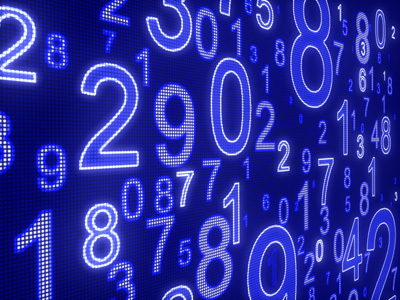Numbers everywhere! In this quiz we look at 3-figure ones.

# Addition and Subtraction 3 (Medium)

So, you have made it to the third quiz in our Medium section of Eleven Plus maths quizzes on Addition and Subtraction. If you have already played all our previous quizzes on the topic, then well done you! If you haven’t then you may wish to go back and play them before you tackle this quiz.

Solving addition and subtraction problems is one of the easiest parts of maths. But what if the questions involve 3-figure numbers in the hundreds? You will be pleased to hear that they are no different to any other addition or subtraction problems – they just have an extra column.

TIP: With 3-figure numbers, add the hundreds first, then the tens and then the ones.

Sometimes you will spot some shortcuts which mean you don’t have to do the calculations the long way round. You’ll find more tips in the helpful comments to this quiz. So, if you are ready, let’s play!

1.
620 + 123 - 83 = ?
663
660
657
654
In multiple choice questions, the last digit in the answers can help you find the correct answer more quickly. 0 + 3 - 3 = 0 so you know the correct answer will end with a zero. Be on the LOOKOUT FOR SHORT CUTS like this
2.
420 - 403 - 100 + 349 = ?
246
256
266
276
Always look out for shortcuts: the 400s in 420 – 403 cancel each other out, making the answer the same as 20 - 3
3.
751 + 226 - 805 = ?
162
77
177
172
Remember: in multiple choice quizzes, the last digit in a number can show you the right answer. 1 + 6 - 5 = 2, so the last digit in the correct answer will also be a 2
4.
690 + 426 - 888 = ?
218
228
238
248
A quick way to add 690 is to add 700 then subtract 10.
Here’s the other way to solve the problem, by adding the hundreds first, then the tens and then the ones:
690 + 426 - 888 = (600 + 400 - 800) + (90 + 20 - 80) + (0 + 6 - 8) = 200 + 30 + -2 = 228
5.
53 - 467 + 643 = ?
229
228
227
226
To avoid working with negative numbers, you can rearrange the problem:
53 - 467 + 643 = (53 + 643) - 467 = 696 - 467 = 229
6.
353 - 28 + 11 + 27 = ?
362
363
364
365
It's a good idea to add the hundreds first, then the tens and finally the ones:
353 - 28 + 11 + 27 = (300) + (50 + 10 + 20 - 20) + (3 + 1 + 7 - 8) = 300 + 60 + 3 = 363
7.
600 + 300 + 400 - 678 = ?
626
624
622
620
600 + 400 = 1,000. Keep your eyes peeled, looking for ways to break the problem up into smaller sums
8.
909 + 76 - 654 - 13 = ?
318
320
322
324
TIP: With numbers less than 1,000, add the hundreds first, then the tens and then the ones.
909 + 76 - 654 - 13 = (900 - 600) + (0 + 70 - 50 - 10) + (9 + 6 - 4 - 3) = 300 + 10 + 8 = 318
You can repeat the procedure if you are having difficulty in doing it in your head
9.
894 - 183 - 500 + 17 = ?
208
216
211
228
Remember, 100s first, then 10s, then ones:
894 - 183 - 500 + 17 = (800 - 500 - 100) + (90 + 10 - 80 - 0) + (4 + 7 - 3 - 0) = 200 + 20 + 8 = 228
10.
926 + 220 - 403 = ?
743
703
783
683
With numbers less than 1,000, add the hundreds first, then the tens and then the ones.
926 + 220 - 403 = (900 + 200 - 400) + (20 + 20 - 0) + (6 + 0 - 3) = (700) + (40) + (3) = 743
You can ignore the zeros
Author:  Frank Evans